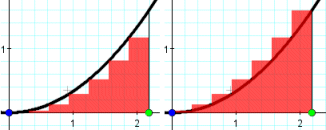Go to contentThe left hand rule is an approximate method for finding the area under the curve f(x) between the limits x=a and x = b which uses the formula:
\qquad \displaystyle \int_a^b f(x) \, dx = h(f(x_0) + f(x_1) + ... + f(x_{n-1}))
where x_0, x_1,... x_{n-1} \, are the values of x at the left hand end of n strips, each of width hYou can get a better display of the maths by downloading special TeX fonts from jsMath. In the meantime, we will do the best we can with the fonts you have, but it may not be pretty and some equations may not be rendered correctly.

## Glossary

### rule

A method for connecting one value with another.

### union

The union of two sets A and B is the set containing all the elements of A and B.

Full Glossary List

## This question appears in the following syllabi:

SyllabusModuleSectionTopicExam Year
AP Calculus AB (USA)4IntegrationApproximate integration-
AP Calculus BC (USA)4IntegrationApproximate integration-
Methods (UK)M9IntegrationApproximate integration-
OCR A-Level (UK - Pre-2017)FP2IntegrationApproximate integration-